# Add new rows and columns to Pandas dataframe

We often get into a situation where we want to add a new row or column to a dataframe after creating it. A quick and dirty solution which all of us have tried atleast once while working with pandas is re-creating the entire dataframe once again by adding that new row or column in the source i.e. csv, txt, DB etc. Pandas is a feature rich Data Analytics library and gives lot of features to achieve these simple tasks of add, delete and update. In this post we will see what are the different ways a Pandas user can add a new row or column to a dataframe.

Create a New Dataframe with Sales data from three different region. We have data from following region: West, North and South.

``````import pandas as pd
df = pd.DataFrame({'Region':['West','North','South'],
'Company':['Costco','Walmart','Home Depot'],
'Product':['Dinner Set','Grocery','Gardening tools'],
'Month':['September','July','February'],
'Sales':[2500,3096,8795]})
df
``````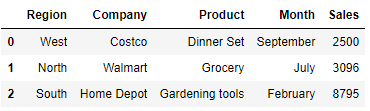Let’s see how to add the data for one region i.e. East in this dataframe. Lets add this region as a separate new row in the dataframe.

New Data Row for East Region:

This is a data dictionary with the values of one Region - East that we want to enter in the above dataframe. The data is basically a list with Dictionary having column as key and their corresponding values.

``````data = [{'Region':'East','Company':'Shop Rite','Product':'Fruits','Month':'December','Sales': 1265}]
``````

We would be using Dataframe Append function to add this data for Region-East into the existing dataframe.

How does Pandas Append Function works?

It basically creates a new dataframe object with the new data row at the end of the dataframe. The old dataframe will be unchanged.

``````df.append(data,ignore_index=True,sort=False)
``````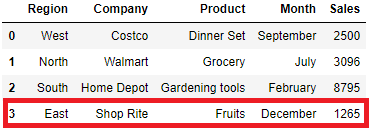`ignore_index` will set the index label if set True, You can see the new row inserted having index value as 3. The default sorting is deprecated and will change to not-sorting in a future version of pandas.

If `sort` set to True then columns will be sorted in alphabetical order and if column starts with numbers then those columns will be first followed by the columns that doesn’t start with numbers and sorted alpabetically.

``````df.append(data,ignore_index=True,sort=True)
``````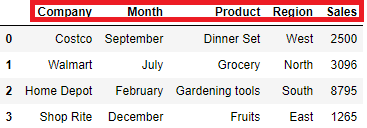## Dataframe loc to Insert a row

`loc` is used to access a group of rows and columns by labels or a boolean array. However with an assignment(=) operator you can also set the value of a cell or insert a new row all together at the bottom of the dataframe

``````df.loc=list(data.values())
or
df.loc[len(df.index)]=list(data.values())
df
``````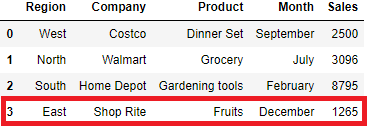## Dataframe iloc to update row at index position

We can replace a row with the new data as well using `iloc`, which is integer-location based indexing for selection by position. In our original dataframe we want to replace the data for North Region with the new data of East Region. Update the row at index position 1 using `iloc` and `list` values of the data dictionary i.e.

``````list(data.values()) = ['East','Shop Rite','Fruits','December',1265]
``````

Update row at Index position 1

``````df.iloc=list(data.values())
df
``````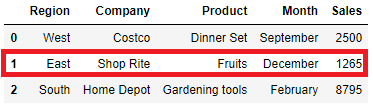So far we have seen how to add the new row at the bottom of the dataframe or replace an existing row with the new one. In the following section we will see how to add a new row in between two rows of a dataframe.

## Insert row at specific Index Position

In our original dataframe we will add the new row for east region at position 2 i.e. insert this new row at second position and the existing row at index 1,2 will cut over to index 2,3

``````data = pd.DataFrame({'Region':'East','Company':'Shop Rite','Product':'Fruits','Month':'December','Sales': 1265}, index=[0.5])
df = df.append(data, ignore_index=False)
df = df.sort_index().reset_index(drop=True)
df
``````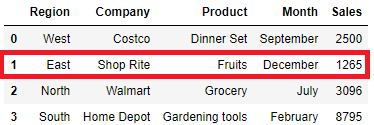## Dataframe append to add New Row

You can also add a new row as a dataframe and then append this new row to the existing dataframe at the bottom of the original dataframe. This is a quick solution when you want to do keep the new record separately in a different dataframe and after some point in time you need to merge that together.

``````b = pd.DataFrame.from_dict(data)
b
``````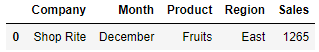Add this new dataframe of new row into our existing original dataframe(df)

``````df.append(b,sort=False)
``````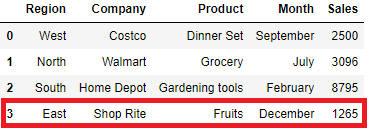## Add New Column to Dataframe

Pandas allows to add a new column by initializing on the fly. For example: the list below is the purchase value of three different regions i.e. West, North and South. We want to add this new column to our existing dataframe above

``````purchase = [3000, 4000, 3500]

df.assign(Purchase=purchase)
``````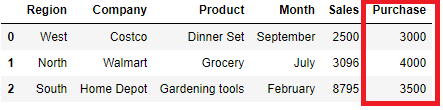## Add Multiple Column to Dataframe

Lets add these three list (Date, City, Purchase) as column to the existing dataframe  using `assign` with a dict of column names and values

``````Date = ['1/9/2017','2/6/2018','7/12/2018']
City = ['SFO', 'Chicago', 'Charlotte']
Purchase = [3000, 4000, 3500]

df.assign(**{'City' : City, 'Date' : Date,'Purchase':Purchase})
``````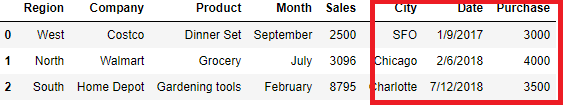Tags:

Categories:

Updated: